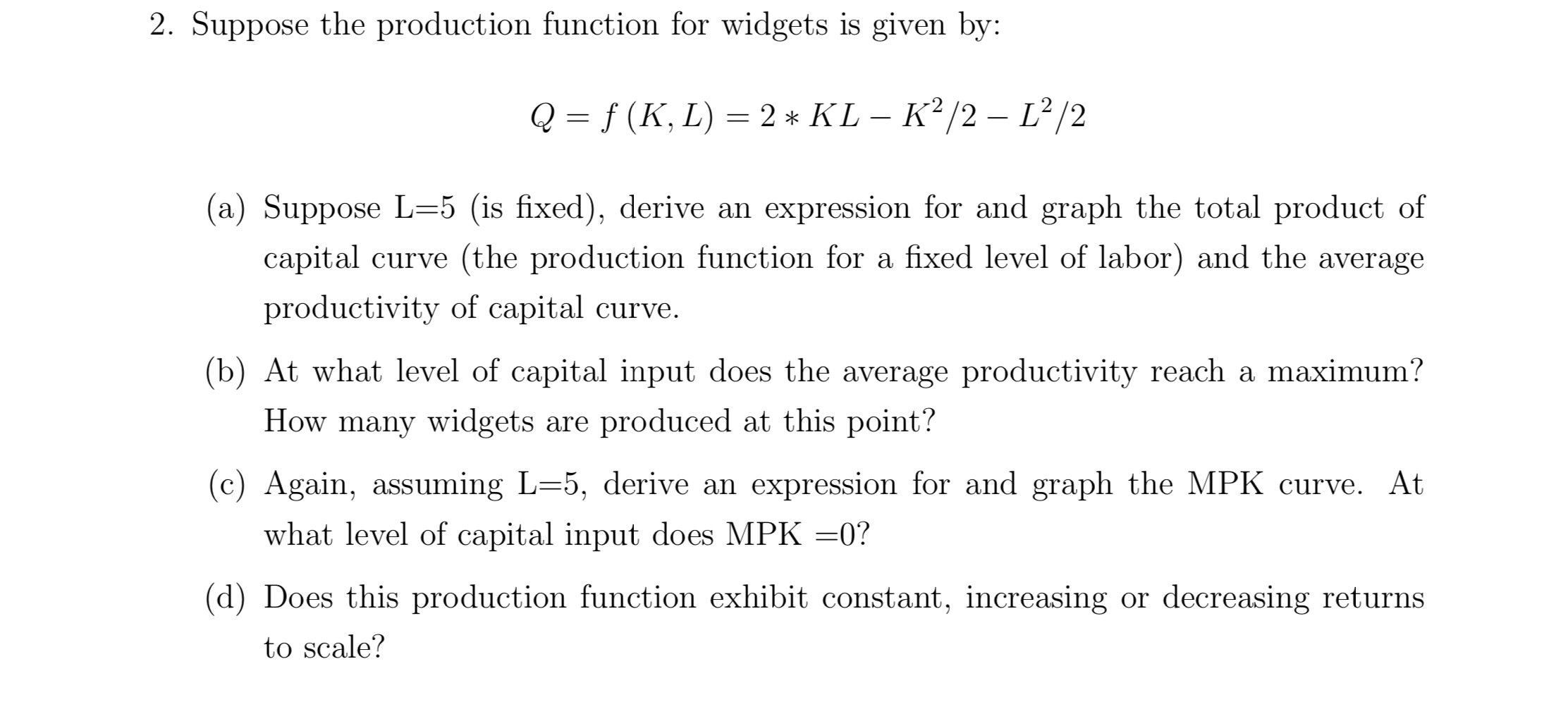# 2. Suppose the production function for widgets is given by:f (K, L) 2* KL - K2/2- L2/2Q1(a) Suppose L-5 (is fixed), derive an expression for and graph the total product ofcapital curve (the production function for a fixed level of labor) and the averageproductivity of capital curve.(b) At what level of capital input does the average productivity reach a maximum?How many widgets are produced at this point?(c) Again, assuming L=5, derive an expression for and graph the MPK curve. Atwhat level of capital input does MPK =0?(d) Does this production function exhibit constant, increasing or decreasing returnsto scale?

Question
1 viewshelp_outlineImage Transcriptionclose2. Suppose the production function for widgets is given by: f (K, L) 2* KL - K2/2- L2/2 Q 1 (a) Suppose L-5 (is fixed), derive an expression for and graph the total product of capital curve (the production function for a fixed level of labor) and the average productivity of capital curve. (b) At what level of capital input does the average productivity reach a maximum? How many widgets are produced at this point? (c) Again, assuming L=5, derive an expression for and graph the MPK curve. At what level of capital input does MPK =0? (d) Does this production function exhibit constant, increasing or decreasing returns to scale? fullscreen
check_circle

Step 1

*  Hello. Since your question has multiple sub-parts, we will solve first three sub-parts for you. If you want remaining sub-parts to be solved, then please resubmit the whole question and specify those sub-parts you want us to solve.

(a) When L = 5 total product of capital is same as the production function with fixed level of labor equal to 5 units.

TP = 2*K*5 - K2 / 2 - 52 / 2

= 10 K - K2 / 2 - 25/ 2

The TP curve is shown below:

Step 2

AP =  TP / K

= 10 - K /2 - 25 / 2K

The AP curve is shown below:

Step 3

(b) The level of capital that maximizes AP is:

dAP / dK = - 1/2 - 25 / 2K2

dAP / dK = 0

- 1/2 - 25 / 2K2 = 0

K = 5

(we have got value of K as -5 or +5, we take only +5 as K can never be negative)

Thus, at K=5 average productivity of capital reach its maximum.

No...

### Want to see the full answer?

See Solution

#### Want to see this answer and more?

Solutions are written by subject experts who are available 24/7. Questions are typically answered within 1 hour.*

See Solution
*Response times may vary by subject and question.
Tagged in